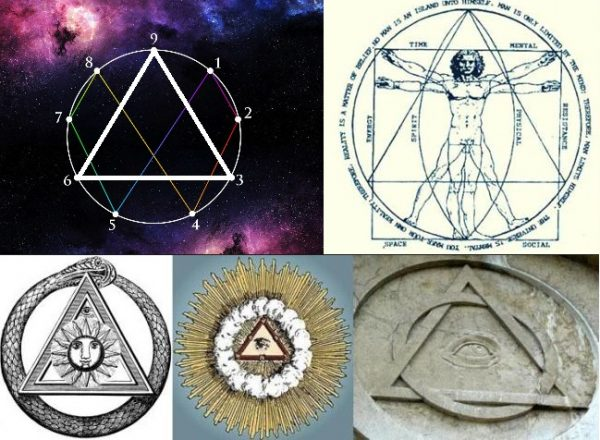# Something Incredible Happens When You Start the Fibonacci Sequence with a 3

The fibonacci sequence is a number pattern which occurs when you start with 0 and 1, and continue to add the subsequent numbers. So-

0+1=1

1+1=2

1+2=3

2+3=5

3+5=8

And so on- so we are just adding the last 2 digits in the sequence. 0 1 1 2 3 5 8 13 21 34 55 89 144 and so on. What this does is (after the first few numbers in the sequence) is it creates a ratio close to 1.618- also known as the golden ratio.

This ratio can be witnessed in everything such as the human anatomy, plants, flowers, the universe itself and even successful companies are well known to use this ratio in the design of their products and logos. If god or your representation of what you would call a creator was a number, that number would be 1.618.

3

3

6

9

15

24

39

63

So you may ask what is so magical about this sequence and the first clue starts with the number 33, as 33 is the start of this sequence. From a numerological standpoint 33 is incredibly powerful. 3 is mind, body and spirit, with 33 representing this in the physical and spiritual realm. 3+3 also makes 6 which is god or the creator. But what really triggered this code for me was the fact that we can derive the number sequence 3-6-9 from 33, as we have the obvious 3, but also 3+3 is 6 and 3×3 is 9. 3-6-9 represent ascension, which is the act of rising into an important position, but 3-6-9 has a lot more than just a spiritual meaning.

“If you only knew the magnificence of the 3, 6 and 9, then you would have a key to the universe.”

~ Nikola Tesla

Back to the fibonacci starting with 33- if we take the first 16 digits of the code we will see that something magica starts to happen-

3

3

6

9

15 1+5=6

24 2+4=6

39 3+9=12 1+2=3

63 6+3=9

Then the code starts again

102 1+0+2=3

165 1+6+5=12 1+2=3

267 2+6+7=15 1+5=6

432 4+3+2=9

699 6+9+9=24 2+4=6

1131 1+1+3+1=6

1830 1+8+3+0=12 1+2=3

2961 2+9+6+1=18 1+8=9

And this repeats infinitely with the code of

3

3

6

9

6

6

3

9

So all numbers within this code are 3, 6 or 9. Once you map out these 3 numbers on a 9 pointed grid like the one below you get part of a code and the shape of a triangle.The second part of the code being binary-

1

2

4

8

16 1+6=7

32 3+2=5

64 6+4=10 1+0=1

128 1+2+8=11 1+1=2

256 2+5+6=13 1+3=4

512 5+1+2=8

1024 1+0+2+4=7

2048 2+0+4+8=14 1+4=5

Again giving the infinite code of 1, 2, 4, 8, 7, 5. The combination of these 2 codes is depicted in the work of Leonardo Da Vinci’s Vitruvian Man. See image above. This is the code Tesla thought could be the solution to creating free energy.

If we look back at the fibonacci code starting with 3- the 12th number is 432. From an article from Collective Evolution “It is said that 432 Hz vibrates with the universe’s golden mean, Phi, and unifies the properties of light, time, space, matter, gravity, and magnetism with biology, the DNA code, and consciousness. When our atoms and DNA start to resonate in harmony with the spiralling pattern of nature, our sense of connection to nature is said to be magnified. The number 432 is also reflected in ratios of the sun, Earth, and moon, as well as the procession of the equinoxes, the Great Pyramid of Egypt, Stonehenge, and the Sri Yantra, among many other sacred sites.”

Fibonacci in itself is a magical sequence and the more you look into it the more it seems to give. Is there a divine blueprint embedded within our number code? I certainly feels that way! Would love to hear what you think on the matter lukemiller@truththeory.com

With recent changes to social media algorithms it is getting more and more difficult for our content to be seen, please share this article to help support independent media!

THIS ARTICLE IS OFFERED UNDER CREATIVE COMMONS LICENSE. IT’S OKAY TO REPUBLISH IT ANYWHERE AS LONG AS ATTRIBUTION BIO IS INCLUDED AND ALL LINKS REMAIN INTACT.